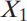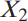×
Log in to StudySoup
Get Full Access to Probability And Statistical Inference - 9 Edition - Chapter 5.3 - Problem 2e
Join StudySoup for FREE
Get Full Access to Probability And Statistical Inference - 9 Edition - Chapter 5.3 - Problem 2e

Already have an account? Login here
×
Reset your password

# Let X1 and X2 be independent random variables withISBN: 9780321923271 41

## Solution for problem 2E Chapter 5.3

Probability and Statistical Inference | 9th Edition

• Textbook Solutions
• 2901 Step-by-step solutions solved by professors and subject experts
• Get 24/7 help from StudySoup virtual teaching assistantsProbability and Statistical Inference | 9th Edition

4 5 1 267 Reviews
22
3
Problem 2E

Problem 2E

Let X1 and X2 be independent random variables with respective binomial distributions b(3, 1/2) and b(5, 1/2). Determine

(a) P(X1 = 2,X2 = 4).

(b) P(X1 + X2 = 7).

Step-by-Step Solution:

Problem 2E

Letandbe independent random variables with respective binomial distributions b(3, 1/2) and b(5, 1/2). Determine

(a) P(= 2,= 4).

(b) P(+= 7).

Step by Step Solution

Step 1 of 3

It is given that X1 and X2 are independent events with binomial distribution b (3, 1/2) and b (5, 1/2).

Apply the binomial distribution, to find the probability.(a) The probability ofSubstitute the values of probability of X equals 2 and 4.Thus, the probability of, 0.05859.

Step 2 of 3

Step 3 of 3

##### ISBN: 9780321923271

Unlock Textbook Solution

Enter your email below to unlock your verified solution to:

Let X1 and X2 be independent random variables with